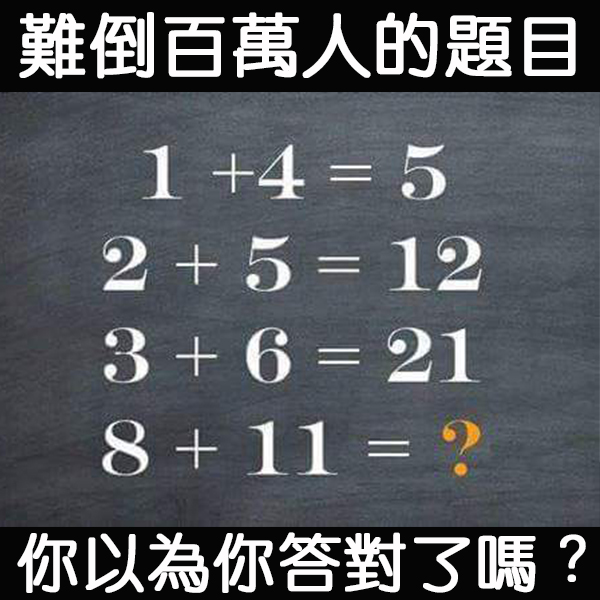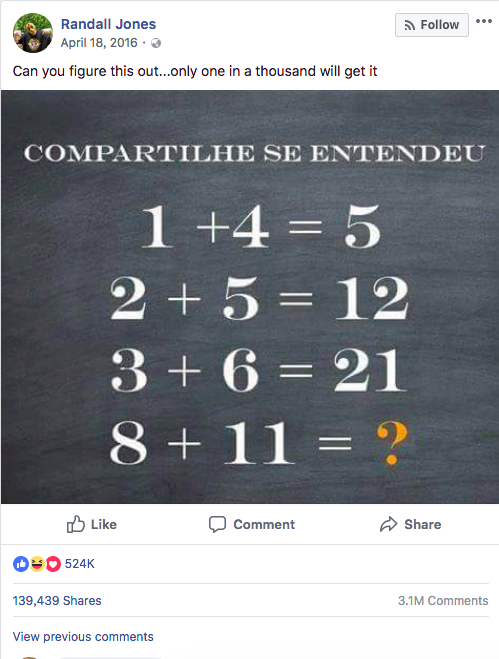1+4=5

5+2+5=12

12+3+6=21

21+8+11= 40

1+(4×1)=5
2+(5×2)=12
3+(6×3)=21

8+(11×8)= 96

1+4=5

5+2+5=12

12+3+6=21

21+4+7=32

32+5+8=45

45+6+9=60

60+7+10=77

77+8+11=?